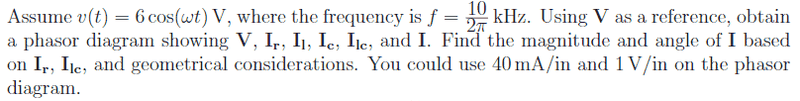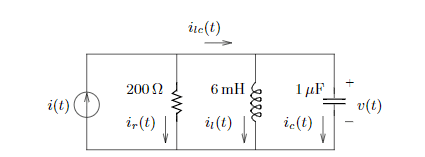# Finding the Current Source of an RLC AC Circuit

YellowBelliedMarmot

## Homework StatementV=IZ[/B]
Zc = 1/(-jwc)
Zl = jwc
w = 2(pi)*f

## The Attempt at a Solution

I'm teaching myself circuit analysis, and it's been rough. Ultimately, I been able to mostly figure everything out, but I've ran into a wall with AC. The main problem I am having is finding i(t), but I don't exactly know how to. I've looked for resources online, but I haven't been able to find any. I'm assuming that I just have to find the impedance of all the components and then use V = IZ to find the current source i(t), but it doesn't seem right. Honestly, I'm not even sure if you can combine impedances in parallel like I did.

Zc = -j100
Zl = j60
Zr = 200

Zeq = (1/200 + 1/(j60) + 1/(-j100))^-1
= 72 + j96

For V, I use the 6cos(wt). I assumed that the voltage would be the same as the voltage across the capacitor due to KVL (pretty sure that I am 100% wrong though).

V = IZ
I = V/Z
i(t) = 6/(72 + j96)
i(t) = .03 - j.04

Now what makes me unsure about what I did is this:

ic(t) = [6cos(wt)]/ [-j100]
= 6/(-j100)
= .06 /_ 90 deg

This solution for ic(t) is not the same as if I did current division:

ic(t) = (.03 - j.04)*(-j100/(200-j40))

So essentially, I have no clue as to what I am doing. Any help would be appreciated, and also any resources that you may know of for help with AC circuit analysis would also be greatly appreciated.

axmls
For V, I use the 6cos(wt). I assumed that the voltage would be the same as the voltage across the capacitor due to KVL

This is correct. Notice that both ends of the voltage source and the capacitor are connected together.

Your first result for ##I_c## is correct. Your second is incorrect because you applied current division incorrectly. With current division, the numerator will be the branch that you're not interested in (try your hand at deriving it for fun). Try it again and see if you get the same result.

Small point: while you do obtain the correct value, you have written your equation for the impedance of a capacitor incorrectly. It should be $$Z_c = \frac{-j}{\omega C}$$ or $$Z_c = \frac{1}{j \omega C},$$ not ##Z_c = 1/(-j \omega C)##. This is because ##1/j = -j##.

•YellowBelliedMarmot
YellowBelliedMarmot
Thank you for pointing out my stupid mistakes! You saved me from going insane!